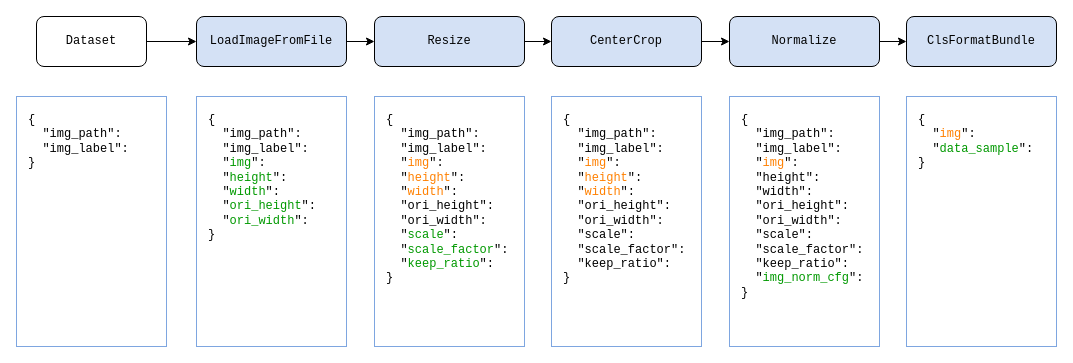Shortcuts

# 数据变换¶

## 数据变换的设计¶

>>> import numpy as np
>>> from mmcv.transforms import Resize
>>>
>>> transform = Resize(scale=(224, 224))
>>> data_dict = {'img': np.random.rand(256, 256, 3)}
>>> data_dict = transform(data_dict)
>>> print(data_dict['img'].shape)
(224, 224, 3)


MMCV 为所有的数据变换类提供了一个统一的基类 (BaseTransform)：

class BaseTransform(metaclass=ABCMeta):

def __call__(self, results: dict) -> dict:

return self.transform(results)

@abstractmethod
def transform(self, results: dict) -> dict:
pass


## 数据流水线¶pipeline = [
dict(type='Resize', size=256, keep_ratio=True),
dict(type='CenterCrop', crop_size=224),
dict(type='Normalize', mean=[123.675, 116.28, 103.53], std=[58.395, 57.12, 57.375]),
dict(type='ClsFormatBundle')
]

dataset = dict(
...
pipeline=pipeline,
...
)


## 常用的数据变换类¶

### 数据加载¶

class 功能
LoadImageFromFile 根据路径加载图像
LoadAnnotations 加载和组织标注信息，如 bbox、语义分割图等

### 数据预处理及增强¶

class 功能
Pad 填充图像边缘
CenterCrop 居中裁剪
Normalize 对图像进行归一化
Resize 按照指定尺寸或比例缩放图像
RandomResize 缩放图像至指定范围的随机尺寸
RandomMultiscaleResize 缩放图像至多个尺寸中的随机一个尺寸
RandomGrayscale 随机灰度化
RandomFlip 图像随机翻转
MultiScaleFlipAug 支持缩放和翻转的测试时数据增强

### 数据格式化¶

class 功能
ToTensor 将指定的数据转换为 torch.Tensor
ImageToTensor 将图像转换为 torch.Tensor

## 自定义数据变换类¶

import random
import mmcv
from mmcv.transforms import BaseTransform, TRANSFORMS

@TRANSFORMS.register_module()
class MyFlip(BaseTransform):
def __init__(self, direction: str):
super().__init__()
self.direction = direction

def transform(self, results: dict) -> dict:
img = results['img']
results['img'] = mmcv.imflip(img, direction=self.direction)
return results


import numpy as np

transform = MyFlip(direction='horizontal')
data_dict = {'img': np.random.rand(224, 224, 3)}
data_dict = transform(data_dict)
processed_img = data_dict['img']


pipeline = [
...
dict(type='MyFlip', direction='horizontal'),
...
]


## 变换包装¶

### 字段映射（KeyMapper）¶

pipeline = [
...
dict(type='KeyMapper',
mapping={
'img': 'gt_img',  # 将 "gt_img" 字段映射至 "img" 字段
},
auto_remap=True,  # 在完成变换后，将 "img" 重映射回 "gt_img" 字段
transforms=[
# 在 RandomFlip 变换类中，我们只需要操作 "img" 字段即可
dict(type='RandomFlip'),
])
...
]


### 随机选择（RandomChoice）和随机执行（RandomApply）¶

pipeline = [
...
dict(type='RandomChoice',
transforms=[
[
dict(type='Posterize', bits=4),
dict(type='Rotate', angle=30.)
],  # 第一种随机变化组合
[
dict(type='Equalize'),
dict(type='Rotate', angle=30)
],  # 第二种随机变换组合
],
prob=[0.4, 0.6]  # 两种随机变换组合各自的选用概率
)
...
]


pipeline = [
...
dict(type='RandomApply',
transforms=[dict(type='Rotate', angle=30.)],
prob=0.3)  # 以 0.3 的概率执行被包装的数据变换
...
]


1. 应用于多个字段

假设我们需要将数据变换应用于 "lq" (low-quality) 和 "gt" (ground-truth) 两个字段中的图像上。

pipeline = [
# 分别应用于 "lq" 和 "gt" 两个字段，并将二者应设置 "img" 字段
mapping={'img': ['lq', 'gt']},
# 在完成变换后，将 "img" 字段重映射回原先的字段
auto_remap=True,
# 是否在对各目标的变换中共享随机变量
# 更多介绍参加后续章节（随机变量共享）
share_random_params=True,
transforms=[
# 在 RandomFlip 变换类中，我们只需要操作 "img" 字段即可
dict(type='RandomFlip'),
])
]


在多目标扩展的 mapping 设置中，我们同样可以使用 ... 来忽略指定的原始字段。如以下例子中，被包裹的 RandomCrop 会对字段 "img" 中的图像进行裁剪，并且在字段 "img_shape" 存在时更新剪裁后的图像大小。如果我们希望同时对两个图像字段 "lq""gt" 进行相同的随机裁剪，但只更新一次 "img_shape" 字段，可以通过例子中的方式实现：

pipeline = [
mapping={
'img': ['lq', 'gt'],
'img_shape': ['img_shape', ...],
},
# 在完成变换后，将 "img" 和 "img_shape" 字段重映射回原先的字段
auto_remap=True,
# 是否在对各目标的变换中共享随机变量
# 更多介绍参加后续章节（随机变量共享）
share_random_params=True,
transforms=[
# RandomCrop 类中会操作 "img" 和 "img_shape" 字段。若 "img_shape" 空缺，
# 则只操作 "img"
dict(type='RandomCrop'),
])
]

2. 应用于一个字段的一组目标

假设我们需要将数据变换应用于 "images" 字段，该字段为一个图像组成的 list。

pipeline = [
# 将 "images" 字段下的每张图片映射至 "img" 字段
mapping={'img': 'images'},
# 在完成变换后，将 "img" 字段下的图片重映射回 "images" 字段的列表中
auto_remap=True,
# 是否在对各目标的变换中共享随机变量
share_random_params=True,
transforms=[
# 在 RandomFlip 变换类中，我们只需要操作 "img" 字段即可
dict(type='RandomFlip'),
])
]


#### 装饰器 cache_randomness¶

TransformBroadcaster 中，我们提供了 share_random_params 选项来支持在多次数据变换中共享随机状态。例如，在超分辨率任务中，我们希望将随机变换同步作用于低分辨率图像和原始图像。如果我们希望在自定义的数据变换类中使用这一功能，需要在类中标注哪些随机变量是支持共享的。这可以通过装饰器 cache_randomness 来实现。

from mmcv.transforms.utils import cache_randomness

@TRANSFORMS.register_module()
class MyRandomFlip(BaseTransform):
def __init__(self, prob: float, direction: str):
super().__init__()
self.prob = prob
self.direction = direction

@cache_randomness  # 标注该方法的输出为可共享的随机变量
def do_flip(self):
flip = True if random.random() > self.prob else False
return flip

def transform(self, results: dict) -> dict:
img = results['img']
if self.do_flip():
results['img'] = mmcv.imflip(img, direction=self.direction)
return results


#### 装饰器 avoid_cache_randomness¶

from mmcv.transforms.utils import avoid_cache_randomness

@TRANSFORMS.register_module()
@avoid_cache_randomness
class MyRandomTransform(BaseTransform):

def transform(self, results: dict) -> dict:
...


avoid_cache_randomness 标记的数据变换类，当其实例被 TransformBroadcaster 包装且将参数 share_random_params 设置为 True 时，会抛出异常，以此提醒用户不能这样使用。

1. avoid_cache_randomness 只用于装饰数据变换类（BaseTransfrom 的子类），而不能用与装饰其他一般的类、类方法或函数

2. avoid_cache_randomness 修饰的数据变换作为基类时，其子类将不会继承这一特性。如果子类仍无法共享随机变量，则应再次使用 avoid_cache_randomness 修饰

3. 只有当一个数据变换具有随机性，且无法共享随机参数时，才需要以 avoid_cache_randomness 修饰。无随机性的数据变换不需要修饰

© Copyright 2018-2022, OpenMMLab. Revision 98e7b3ab.

Built with Sphinx using a theme provided by Read the Docs.
Versions
latest
stable
2.x
v2.0.1
v2.0.0
1.x
v1.7.1
v1.7.0
v1.6.2
v1.6.1
v1.6.0
v1.5.3
v1.5.2_a
v1.5.1
v1.5.0
v1.4.8
v1.4.7
v1.4.6
v1.4.5
v1.4.4
v1.4.3
v1.4.2
v1.4.1
v1.4.0
v1.3.18
v1.3.17
v1.3.16
v1.3.15
v1.3.14
v1.3.13